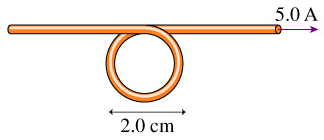# Problem: What is the magnetic field at the center of the loop in the figure? (Figure 1)

###### FREE Expert Solution

Magnetic field:

$\overline{){\mathbf{B}}{\mathbf{=}}\frac{{\mathbf{\mu }}_{\mathbf{0}}\mathbf{i}}{\mathbf{2}\mathbf{\pi }\mathbf{r}}}$

The magnetic field at the center of the loop will be the sum of fields due to current-carrying wire and the circular loop.###### Problem Details

What is the magnetic field at the center of the loop in the figure? (Figure 1)